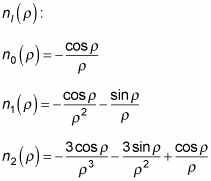##### Quantum Physics For Dummies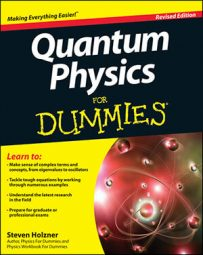In quantum physics, you can apply the spherical Bessel and Neumann functions to a free particle (a particle which is not constrained by any potential). The wave function in spherical coordinates takes this form: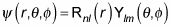and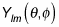gives you the spherical harmonics. The problem is now to solve for the radial part, Rnl(r). Here's the radial equation: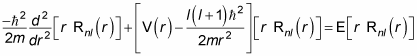For a free particle, V(r) = 0, so the radial equation becomes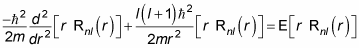The way you usually handle this equation is to substitute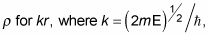and because you have a version of the same equation for each n index it is convenient to simply remove it, so that Rnl (r) becomes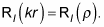This substitution means thatbecomes the following: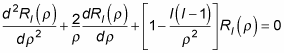The radial part of the equation looks tough, but the solutions turn out to be well-known — this equation is called the spherical Bessel equation, and the solution is a combination of the spherical Bessel functions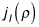and the spherical Neumann functions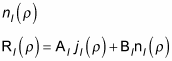where Al and Bl are constants. So what are the spherical Bessel functions and the spherical Neumann functions? The spherical Bessel functions are given by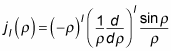Here's what the first few iterations oflook like: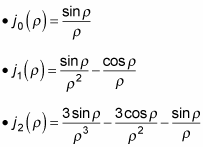How about the spherical Neumann functions? The spherical Neumann functions are given by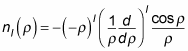Here are the first few iterations of